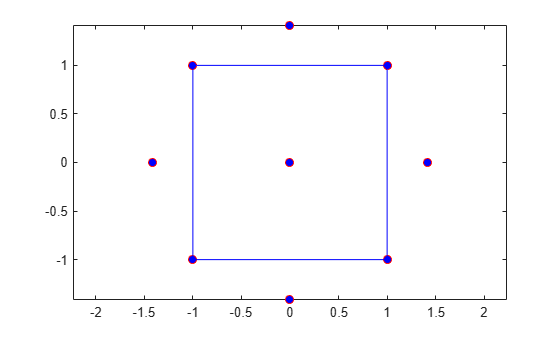Documentation

# ccdesign

Central composite design

## Syntax

```dCC = ccdesign(n) [dCC,blocks] = ccdesign(n) [...] = ccdesign(n,'Name',value) ```

## Description

`dCC = ccdesign(n)` generates a central composite design for `n` factors. `n` must be an integer `2` or larger. The output matrix `dCC` is m-by-`n`, where m is the number of runs in the design. Each row represents one run, with settings for all factors represented in the columns. Factor values are normalized so that the cube points take values between `-1` and `1`.

`[dCC,blocks] = ccdesign(n)` requests a blocked design. The output `blocks` is an m-by-1 vector of block numbers for each run. Blocks indicate runs that are to be measured under similar conditions to minimize the effect of inter-block differences on the parameter estimates.

`[...] = ccdesign(n,'Name',value)` specifies one or more optional name/value pairs for the design. Valid parameters and their values are listed in the following table. Specify `Name` in single quotes.

ParameterDescriptionValuesValue Description
`center`

Number of center points.

Integer

Number of center points to include.

`'uniform'`

Select number of center points to give uniform precision.

`'orthogonal'`

Select number of center points to give an orthogonal design. This is the default.

`fraction`

Fraction of full-factorial cube, expressed as an exponent of 1/2.

`0`Whole design. Default when `n` ≤ 4.
`1`1/2 fraction. Default when
4 < `n` ≤ 7 or `n` > 11.
`2`1/4 fraction. Default when
7 < `n` ≤ 9
`3`1/8 fraction. Default when
`n` = 10.
`4`1/16 fraction. Default when `n` = 11.
`type`

Type of CCD.

`'circumscribed'`

Circumscribed (CCC). This is the default.

`'inscribed'`

Inscribed (CCI).

`'faced'`

Faced (CCF).

`blocksize`

Maximum number of points per block.

Integer

The default is `Inf`.

## Examples

collapse all

Create a 2-factor central composite design.

`dCC = ccdesign(2,'type','circumscribed')`
```dCC = 16×2 -1.0000 -1.0000 -1.0000 1.0000 1.0000 -1.0000 1.0000 1.0000 -1.4142 0 1.4142 0 0 -1.4142 0 1.4142 0 0 0 0 ⋮ ```

The center point is run 8 times to reduce the correlations among the coefficient estimates.

Visualize the design.

```plot(dCC(:,1),dCC(:,2),'ro','MarkerFaceColor','b') X = [1 -1 -1 -1; 1 1 1 -1]; Y = [-1 -1 1 -1; 1 -1 1 1]; line(X,Y,'Color','b') axis square equal```